# c++ 如何销毁外部调用的类

## C++：如何正确的使用接口类

2018-07-02 18:22:05 netyeaxi 阅读数 5591

### 提供接口与实现

``````// circle.h
// 圆的接口类
class Circle {
public:
virtual ~Circle() {};

// 接口方法：面积
virtual double area() = 0;
};``````

``````// circle_impl.h
#include "circle.h"

// 圆的具体实现类
class CircleImpl : public Circle {

private:
public:
double area() override;
};``````
``````// circle_impl.cpp
#include <cmath>
#include "circle_impl.h"

inline double pi() {
return std::atan(1) * 4;
};

};

double CircleImpl::area() {
};``````

``````// circle_manager.h
#include "circle.h"

// 圆的创建工厂类
class CircleManager {
public:
static Circle* create(double radius);     // 创建circle实例
static void destroy(Circle* circlePtr);   // 销毁circle实例
};``````
``````// circle_manager.cpp
#include "circle_manager.h"
#include "circle_impl.h"

return circlePtr;
};

void CircleManager::destroy(Circle* circlePtr) {
delete circlePtr;
}; ``````

``````proj-+
|-inc-+
|     |-circle.h
|     |-circle_manager.h
|
|-src-+
|-circle_impl.h
|-circle_impl.cpp
|-circle_manager.cpp``````

### 如何使用静态库？

1). 把inc目录添加到“附加包含目录”中。

2). “附加依赖项”中添加circle.lib。

3). 把circle.lib所在目录的路径添加到“附加库目录”中。

``````// main.cpp
#include <iostream>
#include "circle_manager.h"
#include "circle.h"

int main()
{
Circle* circlePtr = CircleManager::create(3);
cout << circlePtr->area() <<endl;
CircleManager::destroy(circlePtr);

system("pause");

return 0;
}``````

### 如何编译成动态库？

``````// dll_export.h
// if windows .dll
#ifdef _WINDLL

#ifdef DLL_API_EXPORTS
#define DLL_API __declspec(dllexport)
#else
#define DLL_API __declspec(dllimport)
#endif

// else if Linux or macOS .so
#else
#define DLL_API
#endif``````

windows： /D "DLL_API_EXPORTS" /D "_WINDLL"

Linux： 不用配置额外参数

circle.h和circle_manager.h也要做相应改动：

``````// circle.h
#pragma once
#include "dll_export.h"

// 圆的接口类
class DLL_API Circle
{
public:
virtual ~Circle() {};

// 接口方法：面积
virtual double area() = 0;
};``````
``````// circle_manager.h
#pragma once

#include "circle.h"
#include "dll_export.h"

// 圆的创建工厂类
class DLL_API CircleManager
{
public:
static void destroy(Circle* circlePtr);
};``````

``````proj-+
|-inc-+
|     |-circle.h
|     |-circle_manager.h
|
|-src-+
|     |-circle_impl.h
|     |-circle_impl.cpp
|     |-circle_manager.cpp
|
|-bin-+
|-circle.lib
|-circlr.dll``````

### 如何使用动态库？

1). 代码中添加#pragma comment(lib,"circle.lib")， 这里是circle.lib，不是circle.dll。

2). 把inc目录添加到“附加包含目录”中。

3). “附加依赖项”中添加circle.lib，这里也是circle.lib，不是circle.dll。

4). 把bin目录所在路径添加到”附加库目录“中。

``````#include <iostream>

#include "circle_manager.h"
#include "circle.h"

#pragma comment(lib,"circle.lib")

int main()
{
Circle* circlePtr = CircleManager::create(3);
cout << circlePtr->area() << endl;
CircleManager::destroy(circlePtr);

system("pause");

return 0;
}``````

### 总结

1、为什么CircleManager类即在提供创建实例的方法又要提供销毁实例的方法？

2、对动态库的调用本文是通过隐式调用的方式完成的，对动态库的调用也可以使用显式调用的方式，但由于windows和Linux在使用显式调用时的API是不同的，不好提供统一的代码，所以本文没有举例，以后有机会再单独行文介绍。

### 参考文档

HowTo: Export C++ classes from a DLL

Exporting C++ classes from a DLL

DLLs in Visual C++

Microsoft Visual Studio .NET 2003 Warning C4251

Exporting classes containing std:: objects (vector, map, etc) from a dll

c++ 如何销毁外部调用的类 相关内容

## C++-->类

2016-08-05 15:36:56 kiwi_berrys 阅读数 512

C++类(Class)总结

C++中使用关键字 class 来定义类, 其基本形式如下:

``````class 类名
{
public:
//公共的行为或属性
private:
//公共的行为或属性
};``````

■ 属性: x坐标, y坐标
■ 方法: 1.设置x,y的坐标值; 2.输出坐标的信息。

``````class Point
{
public:
void setPoint(int x, int y);
void printPoint();

private:
int xPos;
int yPos;
};  ``````

1 数据抽象和封装

2 类定义

(1) 类名

(2) 类成员

class People
{
};
sizeof(People) = 1;
(3) 构造函数

(4) 成员函数

3 类定义补充
3.1 可使用类型别名来简化类

``````class People
{
public:
typedef std::string phonenum; //电话号码类型

phonenum phonePub; //公开号码
private:
phonenum phonePri;//私人号码
}; ``````

3.2 成员函数可被重载

3.3 内联函数

（1）直接在类内部定义。
（2）在类内部声明，加上inline关键字，在类外部定义。
（3）在类内部声明，在类外部定义，同时加上inline关键字。注意：此种情况下，内联函数的定义通常应该放在类定义的同一头文件中，而不是在源文件中。这是为了保证内联函数的定义在调用该函数的每个源文件中是可见的。
3.4 访问限制
public，private，protected 为属性/方法限制的关键字。
3.5 类的数据成员中不能使用 auto、extern和register等进行修饰, 也不能在定义时进行初始化

static const int ia= 30;

4 类声明与类定义
4.1 类声明(declare)
class Screen;

void Test1(Screen& a){};
void Test1(Screen* a){};
4.2 类定义(define)

``````class LinkScreen
{
public:
Screen window;
}; //注意，分号不能丢``````
`````` 因为在类定义之后可以接一个对象定义列表，可类比内置类型，定义必须以分号结束：
``````
``````class LinkScreen{ /* ... */ };
class LinkScreen{ /* ... */ } scr1,scr2; ``````

5 类对象

Sales_item item; //编译器分配了足以容纳一个 Sales_item 对象的存储空间。item 指的就是那个存储空间。

6 隐含的 this 指针

6.1 何时使用 this 指针

``````class Screen
{
...
public:
Screen& set(char);
};
Screen& Screen::set(char c)
{
contents[cursor] = c;
return *this;
}``````

7 类作用域

``````class Screen
{
//类的内部
...
};
//类的外部
char Screen::get(index r, index c) const
{
index row = r * width;      // compute the row location
return contents[row + c];   // offset by c to fetch specified character
} ``````
`````` 注意：成员函数的返回类型不一定在类作用域中。可通过 类名::来判断是否是类的作用域，::之前不属于类的作用域，::之后属于类的作用域。例如
``````

Screen:: 之前的返回类型就不在类的作用域，Screen:: 之后的函数名开始到函数体都是类的作用域。

``````class Screen
{
public:
typedef std::string::size_type index;
index get_cursor() const;
};
Screen::index Screen::get_cursor() const   //注意：index前面的Screen不能少
{
return cursor;
} ``````
`````` 该函数的返回类型是 index，这是在 Screen 类内部定义的一个类型名。在类作用域之外使用，必须用完全限定的类型名 Screen::index 来指定所需要的 index 是在类 Screen 中定义的名字。
``````

1 构造函数可以重载

``````class Sales_item;
{
public:
Sales_item(const std::string&);
Sales_item(std::istream&);
Sales_item(); //默认构造函数
};

2 构造函数自动执行
只要创建该类型的一个对象，编译器就运行一个构造函数：
Sales_item item1("0-201-54848-8");
Sales_item *p = new Sales_item(); ``````
`````` 第一种情况下，运行接受一个 string 实参的构造函数，来初始化变量item1。
第二种情况下，动态分配一个新的 Sales_item 对象，通过运行默认构造函数初始化该对象。
``````

3 构造函数初始化式

Sales_item::Sales_item(const string &book): isbn(book), units_sold(0), revenue(0.0)
{ }

3.1 哪种类需要初始化式
const 对象或引用类型的对象，可以初始化，但不能对它们赋值，而且在开始执行构造函数的函数体之前要完成初始化。

``````class ConstRef
{
public:
ConstRef(int ii);
private:
int i;
const int ci;
int &ri;
};
ConstRef::ConstRef(int ii)
{
i = ii;   // ok
ci = ii;  // error
ri = i;   //
}``````
`````` 应该这么初始化：
``````

ConstRef::ConstRef(int ii): i(ii), ci(i), ri(ii) { }
3.2 成员初始化的次序

3.3 初始化式表达式

``Sales_item(const std::string &book, int cnt, double price): isbn(book), units_sold(cnt), revenue(cnt * price) { }``

3.4 类类型的数据成员的初始化式

Sales_item(): isbn(10, ‘9’), units_sold(0), revenue(0.0) {}
3.5 类对象的数据成员的初始化

``````class A
{
public:
int ia;
B b;
};``````
``````A类对象A a;不管a在局部作用域还是全局作用域，b使用B类的默认构造函数来初始化，ia的初始化取决于a的作用域，a在局部作用域，ia不被初始化，a在全局作用域，ia初始化0。
``````

4 默认构造函数

``````class A
{
public:
A(int a=1,char c =''){}
private:
int ia;
char c1;
};``````

4.1 合成的默认构造函数

5 隐式类类型转换
5.1 只含单个形参的构造函数能够实现从形参类型到该类类型的一个隐式转换

``````class A
{
public:
A(int a)
{
ia =a;
}

bool EqualTo(const A& a)
{
return ia == a.ia;
}

private:
int ia;
};

A a(1);
bool bEq = false;
bEq = a.EqualTo(1);//参数为1，实现从int型到A的隐式转换
``````

5.2抑制由构造函数定义的隐式转换

``````class A
{
public:
explicit A(int a )
{
ia =a;
}

bool EqualTo(const A& a)
{
return ia == a.ia;
}

private:
int ia;
};``````
`````` 通常，除非有明显的理由想要定义隐式转换，否则，单形参构造函数应该为 explicit。将构造函数设置为 explicit 可以避免错误。
``````

1 复制构造函数
1.1 几个要点
(1) 复制构造函数

class Peopel
{
public:
Peopel();//默认构造函数
Peopel(const Peopel&);//复制构造函数
~Peopel();//析构函数
};

Peopel a1; Peopel a2 = a1;

Peopel Func(Peopel b){…}
(2)析构函数

(3) 复制控制

(4) 两种初始化形式
C++ 支持两种初始化形式：直接初始化和复制初始化。直接初始化将初始化式放在圆括号中，复制初始化使用 = 符号。

(5)形参和返回值

(6)初始化容器元素

vector svec(5);

(7)构造函数与数组元素

``````Sales_item primer_eds[] = { string("0-201-16487-6"),
string("0-201-54848-8"),
string("0-201-82470-1"),
Sales_item()
};``````

1.2 合成的复制构造函数
(1)合成的复制构造函数

1.3 定义自己的复制构造函数
(1) 只包含类类型成员或内置类型（但不是指针类型）成员的类，无须显式地定义复制构造函数，也可以复制。

``````class Peopel
{
public:
std::string name;
unsigned int id;
unsigned int age;
};``````

(2) 有些类必须对复制对象时发生的事情加以控制。

1.4 禁止复制

2 赋值操作符

(1)重载赋值操作符
Sales_item& operator=(const Sales_item &);
(2)合成赋值操作符

(3)复制和赋值常一起使用

3 析构函数

(1) 何时调用析构函数

(2)何时编写显式析构函数

(3)合成析构函数

`````` 析构函数与复制构造函数或赋值操作符之间的一个重要区别：即使我们编写了自己的析构函数，合成析构函数仍然运行。
``````

1 友元类

``````class Husband
{
public:
friend class Wife;
private:
double money;//钱是老公私有的，别人不能动，但老婆除外
};

class Wife
{
public:
void Consume(Husband& h)
{
h.money -= 10000;//老婆可以花老公的钱
}
};

Husband h;
Wife w;
w.Consume(h);

2 使其他类的成员函数成为友元
class Husband; //1.声明Husband

class Wife //2.定义Wife类
{
public:
void Consume(Husband& h);
};

class Husband //3.定义Husband类
{
public:
friend void Wife::Consume(Husband& h);//声明Consume函数。
private:
double money;//钱是老公私有的，别人不能动，但老婆除外
};

void Wife::Consume(Husband& h) //4.定义Consume函数。
{
h.money -= 10000;//老婆可以花老公的钱
}``````

(1)声明类Husband
(2)定义类Wife，声明Consume函数
(3)定义类Husband
(4)定义Consume函数。

static 成员，有全局对象的作用，但又不破坏封装。
1 static 成员变量
static 数据成员是与类关联的对象，并不与该类的对象相关联。
static 成员遵循正常的公有／私有访问规则。

2 使用 static 成员而不是全局对象有三个优点。
(1) static 成员的名字是在类的作用域中，因此可以避免与其他类的成员或全局对象名字冲突。
(2) 可以实施封装。static 成员可以是私有成员，而全局对象不可以。
(3) 通过阅读程序容易看出 static 成员是与特定类关联的,这种可见性可清晰地显示程序员的意图。

3 static 成员函数

1) static 函数没有 this 指针
2) static 成员函数不能被声明为 const （将成员函数声明为 const 就是承诺不会修改该函数所属的对象）
3) static 成员函数也不能被声明为虚函数

4 static 数据成员
static 数据成员可以声明为任意类型，可以是常量、引用、数组、类类型，等等。
static 数据成员必须在类定义体的外部定义（正好一次）,并且应该在定义时进行初始化。

double Account::interestRate = 0.035;

5 特殊的静态常量整型成员

static const int period = 30;

6 其他
（1）static 数据成员的类型可以是该成员所属的类类型。非 static 成员只能是自身类对象的指针或引用

``````class Screen
{
public:
// ...
private:
static Screen src1; // ok
Screen *src2;       // ok
Screen src3;        // error
}; ``````

（2）非 static 数据成员不能用作默认实参，static 数据成员可用作默认实参

``````class Screen
{
public:
Screen& clear(char = bkground);
private:
static const char bkground = '#';//static const整形变量可以在类内部初始化。
};``````

c++ 如何销毁外部调用的类 相关内容

## C++中的单例模式

2012-04-14 10:08:40 Hackbuteer1 阅读数 206370
单例模式也称为单件模式、单子模式，可能是使用最广泛的设计模式。其意图是保证一个类仅有一个实例，并提供一个访问它的全局访问点，该实例被所有程序模块共享。有很多地方需要这样的功能模块，如系统的日志输出，GUI应用必须是单鼠标，MODEM的联接需要一条且只需要一条电话线，操作系统只能有一个窗口管理器，一台PC连一个键盘。
单例模式有许多种实现方法，在C++中，甚至可以直接用一个全局变量做到这一点，但这样的代码显的很不优雅。 使用全局对象能够保证方便地访问实例，但是不能保证只声明一个对象——也就是说除了一个全局实例外，仍然能创建相同类的本地实例。
《设计模式》一书中给出了一种很不错的实现，定义一个单例类，使用类的私有静态指针变量指向类的唯一实例，并用一个公有的静态方法获取该实例。
单例模式通过类本身来管理其唯一实例，这种特性提供了解决问题的方法。唯一的实例是类的一个普通对象，但设计这个类时，让它只能创建一个实例并提供对此实例的全局访问。唯一实例类Singleton在静态成员函数中隐藏创建实例的操作。习惯上把这个成员函数叫做Instance()，它的返回值是唯一实例的指针。

```class CSingleton
{
private:
CSingleton()   //构造函数是私有的
{
}
static CSingleton *m_pInstance;
public:
static CSingleton * GetInstance()
{
if(m_pInstance == NULL)  //判断是否第一次调用
m_pInstance = new CSingleton();
return m_pInstance;
}
};```

CSingleton* p1 = CSingleton :: GetInstance();
CSingleton* p2 = p1->GetInstance();
CSingleton & ref = * CSingleton :: GetInstance();

```class CSingleton
{
private:
CSingleton()
{
}
static CSingleton *m_pInstance;
class CGarbo   //它的唯一工作就是在析构函数中删除CSingleton的实例
{
public:
~CGarbo()
{
if(CSingleton::m_pInstance)
delete CSingleton::m_pInstance;
}
};
static CGarbo Garbo;  //定义一个静态成员变量，程序结束时，系统会自动调用它的析构函数
public:
static CSingleton * GetInstance()
{
if(m_pInstance == NULL)  //判断是否第一次调用
m_pInstance = new CSingleton();
return m_pInstance;
}
};
```

```class CSingleton
{
private:
CSingleton()   //构造函数是私有的
{
}
public:
static CSingleton & GetInstance()
{
static CSingleton instance;   //局部静态变量
return instance;
}
};```

Singleton singleton = Singleton :: GetInstance();

```class CSingleton
{
private:
CSingleton()   //构造函数是私有的
{
}
public:
static CSingleton * GetInstance()
{
static CSingleton instance;   //局部静态变量
return &instance;
}
};```

```class CSingleton
{
private:
CSingleton()   //构造函数是私有的
{
}
CSingleton(const CSingleton &);
CSingleton & operator = (const CSingleton &);
public:
static CSingleton & GetInstance()
{
static CSingleton instance;   //局部静态变量
return instance;
}
};```

```class Lock
{
private:
CCriticalSection m_cs;
public:
Lock(CCriticalSection  cs) : m_cs(cs)
{
m_cs.Lock();
}
~Lock()
{
m_cs.Unlock();
}
};

class Singleton
{
private:
Singleton();
Singleton(const Singleton &);
Singleton& operator = (const Singleton &);

public:
static Singleton *Instantialize();
static Singleton *pInstance;
static CCriticalSection cs;
};

Singleton* Singleton::pInstance = 0;

Singleton* Singleton::Instantialize()
{
if(pInstance == NULL)
{   //double check
Lock lock(cs);           //用lock实现线程安全，用资源管理类，实现异常安全
//使用资源管理类，在抛出异常的时候，资源管理类对象会被析构，析构总是发生的无论是因为异常抛出还是语句块结束。
if(pInstance == NULL)
{
pInstance = new Singleton();
}
}
return pInstance;
}```

c++ 如何销毁外部调用的类 相关内容

## C++通过引用来传递和返回类对象

2020-01-09 11:07:51 lijian2017 阅读数 43

[例1] 对象引用举例：

``````class book
{
... ...
};
book Alice;
book &Alice_reference = Alice;``````

[例2] 通过引用的方式来传递和返回对象：

``````#include<iostream>
using namespace std;
class book
{
public:
void setprice(double a);
double getprice();
void settitle(char* a);
char * gettitle();
private:
double price;
char * title;
};
void book::setprice(double a)
{
price = a;
}
double book::getprice()
{
return price;
}
void book::settitle(char* a)
{
title = a;
}
char * book::gettitle()
{
return title;
}
void display(book & b)
{
cout<<"The price of "<<b.gettitle()<<" is \$"<<b.getprice()<<endl;
}
book & init(char *t, double p)
{
static book b;
b.settitle(t);
b.setprice(p);
return b;
}
int main()
{
book Alice;
Alice.settitle("Alice in Wonderland");
Alice.setprice(29.9);
display(Alice);
book Harry;
Harry = init("Harry Potter", 49.9);
display(Harry);
return 0;
}``````

The price of Alice in Wonderland is \$29.9
The price of Harry Potter is \$49.9

c++ 如何销毁外部调用的类 相关内容

## C++类的介绍

2018-02-09 16:11:57 EliminatedAcmer 阅读数 11045

### 1.类的定义：

C++一个类定义的形式如下：
class 类名
{
成员列表
};

1.每个类可以没有成员，也可以有多个成员。
2.类成员可以是数据或函数。
3.所有成员必须在类内部声明，一旦类定义完成后，就没有任何其他方式可以再增加或减少成员。

class 类名
{
返回类型   函数名(形参列表)
{
函数体
}
};

class 类名
{
返回类型   函数名(形参列表);
};

函数体

### 2.类成员的访问控制：

class 类名
{
public:
公有的数据成员和成员函数
protected:
保护的数据成员和成员函数
private:
私有的数据成员和成员函数
};

``````class Test
{
public:
void Sum(int a=0,int b=0);
};
void Test::Sum(int a=0,int b=0)
{
cout<<a+b;
}``````

``````class Test
{
public:
void Sum(int a=0,int b=0);
};
void Test::Sum(int a,int b)
{
cout<<a+b;
}``````

### 3.对象的定义和使用：

``Test test1 , test2;``
``class Test test1 , test2;``

``````Test *p;
p = new Test;``````

``delete p;``

``````Test test;
test.Sum();``````

``````Test *p;
p = new Test;
p->Sum();``````

``````Test test, &r = test;
r.Sum();``````

### 4.构造函数与析构函数：

``````class  Test
{
int x = 0;
...
};``````

C++规定构造函数的名字要与类名保持一致，而且不能指定返回类型。请看下面程序：
``````#include <iostream>

using namespace std;

class Test
{
public:
Test ();
Test (int x,int y);
void Sum();
private:
int a,b;
};
Test::Test()
{

}
Test::Test(int x,int y)
{
a=x;
b=y;
}
void Test::Sum()
{
cout<<a+b;
}
int main()
{
Test test(3,4);
test.Sum();
return 0;
}
``````

``Test test;``

``Test test;``

``````#include <iostream>

using namespace std;

class Test
{
public:
Test ();
Test (int x,int y);
void Sum();
private:
int a,b;
};
Test::Test()
{

}
Test::Test(int x,int y):a(x),b(y)
{

}
void Test::Sum()
{
cout<<a+b;
}
int main()
{
Test test(3,4);
test.Sum();
return 0;
}``````

``````#include <iostream>

using namespace std;

class Test
{
public:
Test () {}
Test (int x,int y):a(x),b(y) {}
void Sum();
private:
int a,b;
};

void Test::Sum()
{
cout<<a+b;
}
int main()
{
Test test(3,4);
test.Sum();
return 0;
}``````

``````#include <iostream>

using namespace std;

class Test
{
public:
Test () {}
Test (int x,int y):a(x),b(y) {}
void Sum();
private:
int a,b;
};

void Test::Sum()
{
cout<<a+b;
}
class AnotherTest
{
public:
AnotherTest(int i,int j):test(i,j) {test.Sum();}
private:
Test test;
};
int main()
{
AnotherTest test(3,4);
return 0;
}
``````

``````#include <iostream>

using namespace std;

class Test
{
public:
Test () {}
Test (int x = 0,int y = 0):a(x),b(y) {}
void Sum();
private:
int a,b;
};

void Test::Sum()
{
cout<<a+b;
}

int main()
{
Test test(3);
test.Sum();
return 0;
}``````

``Test (int x = 0,int y):a(x),b(y) {}``

``````Test test1(3,4);
Test test2 = test1;``````

{
函数体
}

``````#include <iostream>

using namespace std;

class Test
{
public:
Test () {}
Test (int x ,int y):a(x),b(y) {}
Test (const Test& t):a(t.a),b(t.b) {}
void Sum();
private:
int a,b;
};

void Test::Sum()
{
cout<<a+b;
}

int main()
{
Test test1(3,4);
Test test2 = test1;
test2.Sum();
return 0;
}
``````

``Test test2(test1);``

``````#include <iostream>
#include <cstring>

using namespace std;

class Test
{
public:
Test (int x,char *ptr)
{
a = x;
p = new char [x];
strcpy(p,ptr);
}
Test (const Test& C)
{
a = C.a;
p = new char [a];
p = C.p;
}
void Print();
private:
int a;
char *p;
};

void Test::Print()
{
int i = 0;
while(p[i] != '\0')
{
cout<<p[i];
i++;
}

}

int main()
{
char p = "test";
Test a(10,p);
Test b(a);
b.Print();
return 0;
}
``````

``````Test (const Test& C)
{
a = C.a;
p = new char [a];
if(p != 0)
strcpy(p,C.p);
}``````

``````#include <iostream>
#include <cstring>

using namespace std;

class Test
{
public:
Test (int x,char *ptr)
{
a = x;
p = new char [x];
strcpy(p,ptr);
}
Test (const Test& C)
{
a = C.a;
p = new char [a];
if(p != 0)
strcpy(p,C.p);
}
~Test()
{
delete (p);
cout<<"p has been destroyed"<<endl;
}
void Print();
private:
int a;
char *p;
};

void Test::Print()
{
int i = 0;
while(p[i] != '\0')
{
cout<<p[i];
i++;
}
cout<<endl;
}

int main()
{
char p = "test";
Test a(10,p);
Test b(a);
b.Print();
return 0;
}
``````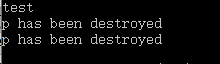### 5.友元机制：

C++提供了友元机制，允许一个类将其非公有成员的访问权限授予指定的函数或类。友元的声明只能在类定义的内部，因此，访问类非公有成员除了自身成员，还有友元。

``````#include <iostream>
#include <cstring>

using namespace std;

class Test
{
public:
Test (int a)
{
x = a;
}
~Test()  //析构函数
{

}
friend void Print(Test& a,Test& b);
private:
int x;
};

void Print(Test& a,Test& b)
{
cout<<a.x*b.x;
}

int main()
{
Test a(10);
Test b(3);
Print(a,b);
return 0;
}
``````

``````#include <iostream>
#include <cstring>

using namespace std;

class B;  //类的前向声明
class A
{
public:
A(){}
~A()  //析构函数
{

}
void Print(B& a);
};

class B
{
public:
B (int a)
{
x = a;
}
private:
int x;
friend void A::Print(B& a);
};
void A::Print(B& a)
{
cout<<a.x;
}
int main()
{
B test1(3);
A test2;
test2.Print(test1);
return 0;
}
``````
类A成功的访问了类B的私有成员，并且打印出来。输出结果为 3。

### 6.继承与派生：

``````#include <iostream>

using namespace std;

class Parallelogram
{
public:
Parallelogram(int a,int b):length(a),width(b) {}
int getLength(){return length;}
int getWidth() {return width;}
private:
int length,width;
};
class Rectangle : public Parallelogram  //公有继承
{
public:
Rectangle(int a,int b):Parallelogram(a,b) {}  //先对基类中的数据成员进行初始化
void Area()     //计算面积
{
cout<<getLength()*getWidth();
}
};
int main()
{
Rectangle r(3,4);
r.Area();
return 0;
}
``````

public(公有继承)，基类中的公有和保护成员保持原属性，私有成员为基类私有。
private(私有继承)，基类中的所有成员在派生类中都是私有的。
protected(保护继承)，基类的公有成员和保护成员在派生类中成了保护成员，私有成员仍为基类私有。

①从基类接收成员，除了构造函数和析构函数，派生类会把全部的成员继承过来，这是没有选择的。
②调整基类成员的访问。
③修改基类成员，可以在派生类中声明一个与基类同名的成员，此操作会覆盖基类的同名成员。
④在定义派生类的时候定义新的成员，定义构造函数和析构函数，初始化的时候必须先将基类的成员初始化(因为并没有继承基类的构造函数)之后才可以对派生类的成员进行初始化。析构函数也一样，需要在派生类中释放基类的数据成员(调用基类的析构函数)。

C++还支持一个派生类同时继承多个基类。

class  派生类名 : 访问标号1 基类名1 , 访问标号2 基类名2 , ....

{

成员列表

}

``````#include <iostream>

using namespace std;

class BaseOne
{
public:
BaseOne() {cout<<"This is BaseOne"<<endl;}
BaseOne(int a):data(a) {cout<<"BaseOne's data is "<<data<<endl;}
private:
int data;
};

class BaseTwo
{
public:
BaseTwo() {cout<<"This is BaseTwo"<<endl;}
BaseTwo(int a):data(a) {cout<<"BaseTwo's data is "<<data<<endl;}
private:
int data;
};

class BaseThree
{
public:
BaseThree() {cout<<"This is BaseThree"<<endl;}
};

class Derive:public BaseOne,public BaseTwo,public BaseThree
{
public:
Derive () {cout<<"This is Derive"<<endl;}
Derive (int a,int b,int c,int d,int e):BaseOne(a),BaseTwo(b),dataOne(c),dataTwo(d),data(e)
{cout<<"Derive's data is "<<data<<endl;}
private:
BaseOne dataOne;
BaseTwo dataTwo;
int data;
};

int main()
{
Derive r1;
cout<<endl;
Derive r2(1,2,3,4,5);
return 0;
}
``````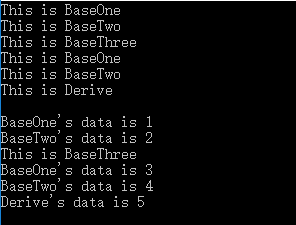``````#include <iostream>
#include <cstring>

using namespace std;

class A
{
public:
void fun() {cout<<"This is A"<<endl;}
};

class B
{
public:
void fun() {cout<<"This is B"<<endl;}
};

class C:public A,public B
{
public:
void hun() {fun();}  //产生二义性
};

int main()
{
C c;
c.hun();
return 0;
}
``````

``void hun() {A::fun(); B::fun();}``

``````#include <iostream>

using namespace std;

class A
{
public:
void fun() {cout<<"This is A"<<endl;}
};

class B:public A
{
public:
void gun() {cout<<"This is B"<<endl;}
};

class C:public A
{
public:
void hun() {cout<<"This is C"<<endl;}
};

class D:public B,public C
{
public:
void kun() {fun();} //产生二义性
};

int main()
{
D d;
d.kun();
return 0;
}
``````

A 是基类，B 是 A 的派生类，C 也是 A 的派生类，而 D 是 B 和 C 的派生类，因此 D 可以访问 A 的数据成员，但现在会产生二义性问题，我们必须显式的指出 fun() 是来自 B 的 还是来自 C 的，但是我们都知道它来自 A , 因此我们希望找到一种方式，使得在继承间接共同基类时只保留一份成员，这就用到了虚基类的机制。

class  派生类名 : virtual  访问标号 虚基类名 , ...

{

成员列表

``class B:virtual public A``

``class C:virtual public A``

``````#include <iostream>

using namespace std;

class A
{
public:
A() {cout<<"This is Grandpa"<<endl;}
A(int a):One(a) {cout<<"Grandpa is "<<One<<" years old"<<endl;}
~A() {cout<<"A is over"<<endl;}
private:
int One;
};

class B:virtual public A
{
public:
B() {cout<<"This is father"<<endl;}
B(int a,int b):A(a),Two(b) {cout<<"father is "<<Two<<" years old"<<endl;}
~B() {cout<<"B is over"<<endl;}
private:
int Two;
};

class C:virtual public A
{
public:
C() {cout<<"This is mother"<<endl;}
C(int a,int b):A(a),Three(b) {cout<<"mother is "<<Three<<" years old"<<endl;}
~C() {cout<<"C is over"<<endl;}
private:
int Three;
};

class D:public B,public C
{
public:
D() {cout<<"This is me"<<endl;}
D(int a,int b,int c,int d):A(a),B(a,b),C(a,c),Four(d) {cout<<"I am "<<Four<<" years old"<<endl;}
~D() {cout<<"D is over"<<endl;}
private:
int Four;
};

int main()
{
D d1;
cout<<endl;
//D d2(65,40,39,13);
return 0;
}
``````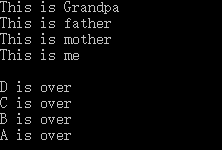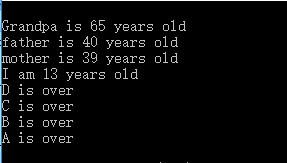### 7.多态性和虚函数：

``````#include <iostream>

using namespace std;

class A
{
public:
void fun() {cout<<"Use A"<<endl;}
};

class B : public A
{
public:
void fun() {cout<<"Use B"<<endl;}
};

int main()
{
B b;
A *p = &b;
p->fun();
return 0;
}
``````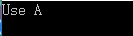``virtual void fun() {cout<<"Use A"<<endl;}``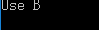virtual   返回类型  函数名 (形式参数列表) = 0;

``````#include <iostream>

using namespace std;

class Sharp
{
public:
virtual double area() = 0;
virtual double volumn() = 0;
};

class Circle : public Sharp
{
public:
Circle(double r):R(r) {}
virtual double area() {return 3.1415926*R*R;}
virtual double volumn() {return 0;}
private:
double R;
};

class Cylinder : public Circle
{
public:
Cylinder(double a,double b):Circle(a),H(b) {}
virtual double volumn() {return area()*H;}
private:
double H;
};

int main()
{
Circle a(20.0);
Cylinder b(10.0,2.0);
cout<<a.area()<<endl;
cout<<b.volumn()<<endl;
return 0;
}
``````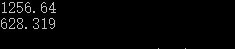c++ 如何销毁外部调用的类 相关内容